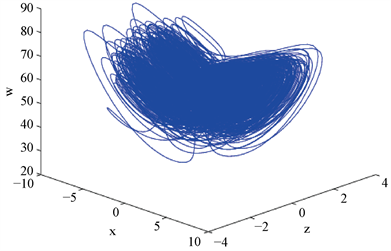#### 理论数学  >> Vol. 10 No. 5 (May 2020)

DOI: 10.12677/PM.2020.105063, PDF, HTML, XML, 下载: 229  浏览: 376

Abstract: The paper investigates the bifurcation of periodic solutions at the zero-zero-Hopf equilibrium of a hyperchaotic Faraday disk dynamo. By means of the averaging theory, the paper obtains the suffi-cient conditions that two periodic solutions will appear at the bifurcation point and discusses the stability of the two orbits.

1. 引言

1996年，Hide等提出了一个self-exciting Faraday圆盘单极发电机，它是一个非线性的三维动力系统 。在此基础上，逐渐发展了很多改进的模型  - ，其中Hide和Moroz提出了一类四维的发电机，它描述了方位涡流(azimuthal eddy currents)的作用。其模型如下：(1.1)

Moroz在文献  中已经做了一些数值分析去研究系统(1.1)的zero-zero-Hopf分支，然而并没有严格的证明。本文将给出系统存在zero-zero-Hopf分支的证明，并把相应的周期解求出来。

2. 超混沌和zero-zero-Hopf分支

${E}_{±}=\left(±{x}_{1},±{x}_{1},±\frac{{x}_{1}}{d},\frac{r{x}_{1}^{2}}{k}\right)$,Figure 1. Hyperchaotic attraction factor of system (1.1)

$\begin{array}{l}{\lambda }^{4}+\left(k+1+d\right){\lambda }^{3}+\left(k+a+c+d+kd-ab-ac\right){\lambda }^{2}\\ +\left(ac+ad+ak+ck+kd-abd-abk-ack\right)\lambda -ka\left(kd-c-d\right)=0\end{array}$ (2.1)

$c=1-b,d=-1,k=0,b<0$ 时，(2.1)有根0，0， $±\omega i$，其中 $\omega =\sqrt{-b}$

$\begin{array}{l}\left(c,d,k\right)=\left(1-b+\epsilon {c}_{1},-1+\epsilon {d}_{1},\epsilon {k}_{1}\right),\text{\hspace{0.17em}}\rho ={c}_{1}+{d}_{1}-b{d}_{1},\\ N={\rho }^{2}+2{d}_{1}\left(a+b-ab\right)\rho +{d}_{1}^{2}\left(b-1\right)\left(b{\left(a-1\right)}^{2}-{a}^{2}\right),\end{array}$

$c=1-b+\epsilon {c}_{1},\text{\hspace{0.17em}}d=-1+\epsilon {d}_{1},\text{\hspace{0.17em}}k=\epsilon {k}_{1},$

$\left\{\begin{array}{l}\stackrel{˙}{x}=a\left(y-x\right),\hfill \\ \stackrel{˙}{y}=\left(b-a\right)x-\left(1-a\right)y+\left(b-1\right)z-xw-{c}_{1}z\epsilon ,\hfill \\ \stackrel{˙}{z}=ax+\left(1-a\right)y+z-{d}_{1}z\epsilon ,\hfill \\ \stackrel{˙}{w}=ra{x}^{2}+r\left(1-a\right)xy-{k}_{1}w\epsilon .\hfill \end{array}$ (2.2)

${\left(x,y,z,w\right)}^{\text{T}}={\left(\epsilon u,\epsilon v,\epsilon p,\epsilon q\right)}^{\text{T}}$。我们把 ${\left(u,v,p,q\right)}^{\text{T}}$ 又表示为 $X={\left(x,y,z,w\right)}^{\text{T}}$，那么系统(2.2)变为

$\begin{array}{c}\stackrel{˙}{X}={F}_{0}\left(t,X\right)+\epsilon {F}_{1}\left(t,X\right)\\ =\left(\begin{array}{c}a\left(y-x\right)\\ \left(b-a\right)x+\left(a-1\right)y+\left(b-1\right)z\\ ax+\left(1-a\right)y+z\\ 0\end{array}\right)-\epsilon \left(\begin{array}{c}0\\ {c}_{1}z+xw\\ {d}_{1}z\\ {k}_{1}w-ra{x}^{2}+r\left(a-1\right)xy\end{array}\right).\end{array}$ (2.3)

$\stackrel{˙}{X}={F}_{0}\left(t,X\right),\text{\hspace{0.17em}}\text{\hspace{0.17em}}{X}_{0}=\left({x}_{0},{y}_{0},{z}_{0},{w}_{0}\right).$ (2.4)

$\begin{array}{l}m=\frac{b\left(1-a\right){x}_{0}+a{y}_{0}+a\left(1-b\right){z}_{0}}{b},\\ x\left(t\right)=\left(ab{x}_{0}-a{y}_{0}+a\left(b-1\right){z}_{0}\right)\frac{\mathrm{cos}\left(\omega t\right)}{b}++\omega a\left({x}_{0}-{y}_{0}\right)\frac{\mathrm{sin}\left(\omega t\right)}{b}+m,\end{array}$

$\begin{array}{l}y\left(t\right)=\left(b\left(a-1\right){x}_{0}+\left(b-a\right){y}_{0}+\left(b-1\right)a{z}_{0}\right)\frac{\mathrm{cos}\left(\omega t\right)}{b}+\left(\left(b-a\right){x}_{0}+\left(a-1\right){y}_{0}+\left(b-1\right){z}_{0}\right)\frac{\mathrm{sin}\left(\omega t\right)}{\omega }+m,\\ z\left(t\right)=\left(b\left(1-a\right){x}_{0}+a{y}_{0}+\left(a+b-ab\right){z}_{0}\right)\frac{\mathrm{cos}\left(\omega t\right)}{b}+\left(a{x}_{0}+\left(1-a\right){y}_{0}+{z}_{0}\right)\frac{\mathrm{sin}\left(\omega t\right)}{\omega }-m,\\ w\left(t\right)={w}_{0}.\end{array}$

${X}_{0}\ne 0$ 时，系统(2.4)的所有解 $X\left(t,{X}_{0}\right)$ 是周期的，其中周期为 $T=\frac{2\pi }{\omega }$。沿着一个周期解 $X\left(t,{X}_{0}\right)$

${M}_{{X}_{0}}\left(t\right)=\frac{1}{b}\left(\begin{array}{cccc}{a}_{11}& {a}_{12}& {a}_{13}& 0\\ {a}_{21}& {a}_{22}& {a}_{23}& 0\\ {a}_{31}& {a}_{32}& {a}_{33}& 0\\ 0& 0& 0& b\end{array}\right),$

$\begin{array}{l}{a}_{11}=ab\mathrm{cos}\left(\omega t\right)+\omega a\mathrm{sin}\left(\omega t\right)+b\left(1-a\right),\\ {a}_{12}=-a\mathrm{cos}\left(\omega t\right)-\omega a\mathrm{sin}\left(\omega t\right)+a,\\ {a}_{13}=a\left(b-1\right)\left(\mathrm{cos}\left(\omega t\right)-1\right),\\ {a}_{21}=\left(a-1\right)b\mathrm{cos}\left(\omega t\right)+\omega \left(a-b\right)\mathrm{sin}\left(\omega t\right)+b\left(1-a\right),\\ {a}_{22}=\left(b-a\right)\mathrm{cos}\left(\omega t\right)+\omega \left(1-a\right)\mathrm{sin}\left(\omega t\right)+a,\end{array}$

$\begin{array}{l}{a}_{23}=\left(b-1\right)\left(a\mathrm{cos}\left(\omega t\right)-\omega \mathrm{sin}\left(\omega t\right)-a\right),\\ {a}_{31}=b\left(1-a\right)\mathrm{cos}\left(\omega t\right)-\omega a\mathrm{sin}\left(\omega t\right)-b\left(1-a\right),\\ {a}_{32}=a\mathrm{cos}\left(\omega t\right)-\omega \left(1-a\right)\mathrm{sin}\left(\omega t\right)-a,\\ {a}_{33}=\left(b+a-ab\right)\mathrm{cos}\left(\omega t\right)-\omega \mathrm{sin}\left(\omega t\right)+a\left(b-1\right).\end{array}$

$G\left({X}_{0}\right)=\frac{1}{T}{\int }_{0}^{T}{M}_{{X}_{0}}^{-1}\left(t\right){F}_{1}\left(t,X\left(t,{x}_{0}\right)\right)\text{d}t=\frac{1}{2{b}^{2}}\left({G}_{1}\left({X}_{0}\right),{G}_{2}\left({X}_{0}\right),{G}_{3}\left({X}_{0}\right),{G}_{4}\left({X}_{0}\right)\right),$

$\begin{array}{l}{G}_{1}\left({X}_{0}\right)=2ab\left(2a-1\right){x}_{0}{w}_{0}-{a}^{2}\left(b+3\right){y}_{0}{w}_{0}-3{a}^{2}\left(1-b\right){z}_{0}{w}_{0}-\left(\left(4a-3\right)\rho +a{d}_{1}\left(b-1\right)\right)ab{x}_{0}\\ \text{ }\text{ }\text{ }\text{ }+\left(3a\rho +b{c}_{1}\left(a-1\right)\right)a{y}_{0}-a\left(\left(3ab-3a-b\right)\rho +{c}_{1}b\right){z}_{0},\\ {G}_{2}\left({X}_{0}\right)=ab\left(4a-b-3\right){x}_{0}{w}_{0}-a\left(ab+3a-2b\right){y}_{0}{w}_{0}+a\left(b-1\right)\left(3a-b\right){z}_{0}{w}_{0}\\ \text{ }\text{ }\text{ }\text{ }-b\left(a\left(3a-4\right)\rho +{c}_{1}\left({a}^{2}-ab+b\right)\right){x}_{0}+\left(\left(3{a}^{2}-ab+b\right)\rho +ab{c}_{1}\left(a-2\right)\right){y}_{0}\\ \text{ }\text{ }\text{ }\text{ }+\left(b-1\right)\left(\left(ab-3{a}^{2}-b\right)\rho +b{d}_{1}\left(ab-2a-b\right)\right){z}_{0},\end{array}$

$\begin{array}{l}{G}_{3}\left({X}_{0}\right)=ab\left(3-4a\right){x}_{0}{w}_{0}+a\left(ab+3a-b\right){y}_{0}{w}_{0}-3{a}^{2}\left(b-1\right){z}_{0}{w}_{0}\\ \text{ }\text{ }\text{ }\text{ }+\left(4ab\rho \left(a-1\right)+b{d}_{1}\left({a}^{2}b-{a}^{2}-b\right)\right){x}_{0}-\left({\left(a-1\right)}^{2}b{c}_{1}+3{a}^{2}\rho +b{d}_{1}\right){y}_{0}\\ \text{ }\text{ }\text{ }\text{ }-\left(\left(b-3{a}^{2}b+3{a}^{2}\right)\rho -2b{d}_{1}\left(ab-a-b\right)\right){z}_{0},\\ {G}_{4}\left({X}_{0}\right)=br\left(3{a}^{2}b-{a}^{2}-4ab+2b\right){x}_{0}^{2}-{a}^{2}r\left(b-3\right){y}_{0}^{2}+3{a}^{2}r{\left(b-1\right)}^{2}{z}_{0}^{2}-4abr\left(a-1\right){x}_{0}{y}_{0}\\ \text{ }\text{ }\text{ }\text{ }+2abr\left(b-1\right)\left(3a-2\right){x}_{0}{z}_{0}-6{a}^{2}r\left(b-1\right){y}_{0}{z}_{0}-2{k}_{1}{b}^{2}{w}_{0}\end{array}$

$\begin{array}{l}{S}_{0}=\left(0,0,0,0\right),\\ {S}_{1}=\left(\sqrt{\frac{{k}_{1}\rho }{r}},\sqrt{\frac{{k}_{1}\rho }{r}},-\sqrt{\frac{{k}_{1}\rho }{r}},\rho \right),\\ {S}_{2}=\left(-\sqrt{\frac{{k}_{1}\rho }{r}},-\sqrt{\frac{{k}_{1}\rho }{r}},\sqrt{\frac{{k}_{1}\rho }{r}},\rho \right).\end{array}$

${S}_{0}$ 对应奇点 ${E}_{0}=\left(0,0,0,0\right)$。对其他两个解 ${S}_{1}$${S}_{2}$，我们有

$G\left({S}_{1}\right)=G\left({S}_{2}\right)=0,$

$\mathrm{det}\left(\left(\partial G/\partial {X}_{0}\right)\left({S}_{1}\right)\right)=\mathrm{det}\left(\left(\partial G/\partial {X}_{0}\right)\left({S}_{2}\right)\right)=-\frac{a{k}_{1}\rho N}{2{b}^{2}}.$

$\left(b{\lambda }^{2}+{k}_{1}b\lambda +2a{k}_{1}\rho \right)\left(4b{\lambda }^{2}+4b{d}_{1}\lambda -N\right)=0.$ (2.5)

${d}_{1}>0,{k}_{1}>0,a\rho <0,N>0$ 时，方程(2.5)的根都有负实部，这意味着在 ${E}_{0}$ 产生的两个周期解 ${X}_{1}\left(t,\epsilon \right)$${X}_{2}\left(t,\epsilon \right)$ 是稳定的。

3. 结束语

NOTES

*通讯作者。

  Hide, R., Skeldon, A.C. and Acheson, D.J. (1996) A Study of Two Novel Self-Exciting Single-Disk Homopolar Dy-namos: Theory. Proceedings of the Royal Society of London. Series A, 452, 1369-1395. https://doi.org/10.1098/rspa.1996.0070  Hide, R. (1997) Nonlinear Quenching of Current Fluctuations in a Self-Exciting Homopolar Dynamo. Nonlinear Processes in Geophysics, 4, 201-205. https://doi.org/10.5194/npg-4-201-1997  Hide, R. (1997) The Nonlinear Differential Equations Governing a Hierarchy of Self-Exciting Coupled Faraday-Disk Homopolar Dynamos. Physics of the Earth and Planetary Interiors, 103, 281-291. https://doi.org/10.1016/S0031-9201(97)00038-1  Hide, R. (2000) Generic Nonlinear Processes in Self-Exciting Dynamos and the Long-Term Behaviour of the Main Geomagnetic Field, Including Polarity Superchrons. Philosophical Transactions of the Royal Society A, 358, 943-955. https://doi.org/10.1098/rsta.2000.0568  Moroz, I.M., Hide, R. and Soward, A.M. (1998) On Self-Exciting Coupled Faraday Disk Homopolar Dynamos Driving Series Motors. Physica D, 117, 128-144. https://doi.org/10.1016/S0167-2789(97)00305-9  Moroz, I.M. (2001) On the Behavior of a Self-Exciting Faraday Disk Homopolar Dynamo with Battery in the Presence of an External Magnetic Field. International Journal of Bifurcation and Chaos, 11, 1695-1705. https://doi.org/10.1142/S0218127401002948  Moroz, I.M. (2001) Self-Exciting Faraday Disk Homopolar Dynamos. International Journal of Bifurcation and Chaos, 11, 2961-2975. https://doi.org/10.1142/S0218127401004017  Moroz, I.M. (2002) On the Behavior of a Self-Exciting Faraday Disk Homopolar Dynamo with a Variable Nonlinear Series Motor. International Journal of Bifurcation and Chaos, 12, 2123-2135. https://doi.org/10.1142/S0218127402005728  Moroz, I.M. (2005) The Extended Malkus-Robbins Dynamo as a Perturbed Lorenz System. Nonlinear Dynamics, 41, 191-210. https://doi.org/10.1007/s11071-005-2808-x  Hide, R. and Moroz, I.M. (1999) Effects Due to Induced Azi-muthal Eddy Currents in a Self-Exciting Faraday Disk Homopolar Dynamo with a Nonlinear Series Motor. I.: Two Special Cases. Physica D, 134, 287-301. https://doi.org/10.1016/S0167-2789(99)00107-4  Moroz, I.M. (2011) Unstable Periodic Orbits in a Four-Dimensional Faraday Disk Dynamo. Geophysical & Astrophysical Fluid Dynamics, 105, 273-286. https://doi.org/10.1080/03091929.2010.511206  Llibre, J. and Makhlouf, A. (2016) Zero-Hopf Bifurcation in the Generalized Michelson System. Chaos Solitons & Fractals, 89, 228-231. https://doi.org/10.1016/j.chaos.2015.11.013  Euzebio, R.D. and Llibre, J. (2017) Zero-Hopf Bifurcation in a Chua System. Nonlinear Analysis: Real World Applications, 37, 31-40. https://doi.org/10.1016/j.nonrwa.2017.02.002  Candido, M.R. and Llibre, J. (2018) Zero-Hopf Bifurcations in 3-Dimensional Differential Systems with No Equilibria. Mathematics and Computers in Simulation, 151, 54-76. https://doi.org/10.1016/j.matcom.2018.03.008  Cid-Montiel, L., Llibre, J. and Stoica, C. (2014) Zero-Hopf Bifurcation in a Hyperchaotic Lorenz System. Nonlinear Dynamics, 75, 561-566. https://doi.org/10.1007/s11071-013-1085-3  Chen, Y.M. and Liang, H.H. (2017) Zero-Zero-Hopf Bifurcation and Ultimate Bound Estimation of a Generalized Lorenz-Stenflo Hyperchaotic System. Mathematical Methods in the Applied Sciences, 40, 3424-3432. https://doi.org/10.1002/mma.4236